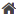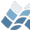NV5 GEOSPATIAL SOFTWARE>  Docs Center  >  Libraries  >  Motley  >  MGH_DENSITY__DEFINE### MGH_DENSITY__DEFINE

MGH_DENSITY__DEFINE

MGH_Density

## Purpose

This class displays a 2-D numeric array as a colour density plot
in a window, with axes and a colour scale. The colour scale can be
edited interactively. The performance of an MGH_DENSITY object
depends on the implementation of the density plot (keyword
IMPLEMENTATION).

## Object Creation Calling Sequence

mgh_new, 'MGH_Density', values[, x, y]

## Positional Parameters

values
A 2D array of numeric data to be plotted
x
Position in the x direction of the columns of data
y
Position in the y direction of the rows of data

## Properties

BYTE_RANGE (Init,Get)
The range of byte values to which the data range is to be mapped.
DATA_RANGE (Init,Get,Set)
The range of data values to be mapped onto the indexed color
range for the density plane and the colour bar. Data values
outside the range are mapped to the nearest end of the range. If
not specified, DATA_RANGE is calculated when the density plane
is created,
STYLE (Init):
The style of the plane. Values are 0 (each data point represented
by a cell of uniform colour) and 1 (colours interpolated between
data points). Default is 0. Note that the value of this property
determines the dimensions that X & Y arrays (if any) must have.
###########################################################################
This software is provided subject to the following conditions:
1. NIWA makes no representations or warranties regarding the
accuracy of the software, the use to which the software may
be put or the results to be obtained from the use of the
software. Accordingly NIWA accepts no liability for any loss
or damage (whether direct of indirect) incurred by any person
through the use of or reliance on the software.
2. NIWA is to be acknowledged as the original author of the
software where the software is used or presented in any form.
###########################################################################